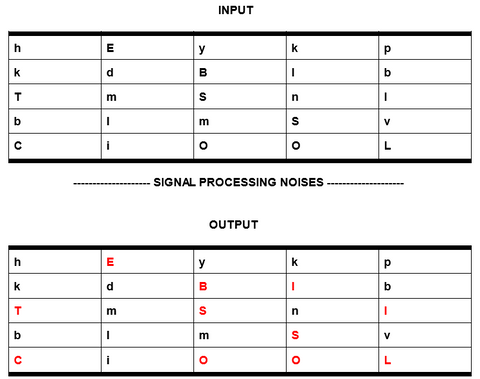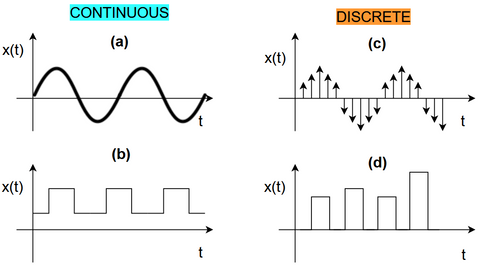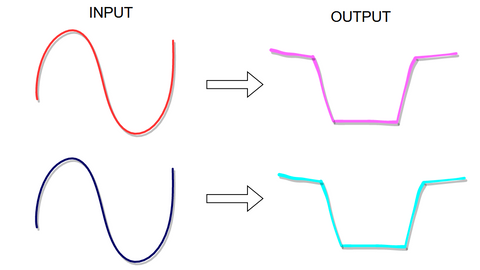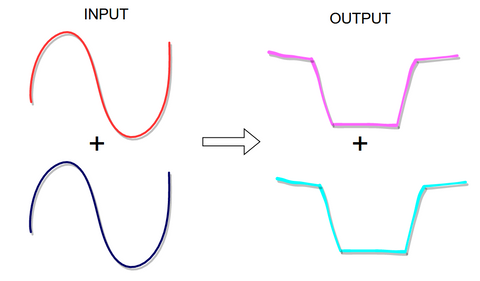# Introduction

So what is this signal processing? Well it can sometimes be like interpreting invisible hieroglyphs! Signal processing refers to the manipulation, analysis, and interpretation of signals in electrical or electronic systems. A signal can be any form of information that conveys meaning, such as sound, images or other data. Signal processing involves mathematical algorithms and techniques used to analyze and modify signals, such as filtering, compression, enhancement and recognition.

Signal processing plays a crucial role in many modern technologies and applications, such as telecommunications, audio and video processing, biomedical engineering, control systems, and computer engineering. In these fields, signals are used to represent and communicate information, and signal processing can be used to extract and isolate this useful information and improve the signal's quality.
An example for this could be removing background noise for a voice recording or smoothing a picture with a lot of unwanted ‘’noise’’. It could also be to decode a somewhat unclear input signal to a clear signal, as we do in the following figure 1.Figure 1: What looks to be random letters turns out to be a hidden message after a little signal processing (red letters).
*Notice that this illustration is just for demonstrating the concept

Figure 1 only works as an illustration of the concept, where the lower case letters are cut off from the input signal to reveal the hidden message. This concept could play out as to cut off certain unwanted frequencies from the input signal. And this is fx. how radios work. A radio catches radio waves which is an electromagnetic wave propagated by an antenna. These radio waves have different frequencies, which each carries the information for different radio channels. By tuning a radio receiver to a specific frequency you can pick up a specific signal and thereby change channels. It’s kinda like decoding invisible hieroglyphs and that is sometimes what signal processing is! To sum up, the goal of signal processing is to extract meaningful information from signals and convert it into a form that is useful for the particular application.

In this blog we will go through some of the fundamental mathematical algorithms and techniques for signal processing. But before you are presented with these fundamental mathematical algorithms and techniques let's boil it down a little.

Often when working with signals for electronics we work in time and frequencies.
So the signals can be viewed in both time and frequency domains. If we represent the transforms in the time domain, we get voltage levels at different times. If we represent the transforms in the frequency domain, we get voltage levels of different frequencies. Therefore situations can arise where we want a certain voltage and therefore a certain frequency and vice versa.

Now the mathematical algorithms and techniques we present to you in this blog are called The Fourier transform, Laplace transform and Z-transform. They are all mathematical tools used for signal processing and analysis. While they share some similarities, they also have distinct differences. Below you’ll see an overview between the similarities and differences for these transforms.

Some words used below are hyperlinks, that will take you to the part where they are explained further

1. ### Domain

The Fourier transform operates on continuous-time signals in the time domain.

The Laplace transform operates on both continuous-time and discrete-time signals.

The Z-transform operates exclusively on discrete-time signals in the time domain.

2. ### Complexity and math

The Fourier transform involves complex exponential function.

The Laplace transform involves complex exponential functions and complex logarithmic functions.

The Z-transform involves complex exponential functions and complex polynomials.

3. ### Stability analysis

Stability analysis is the part of systems and control theory which is used to study and predict the stability or instability characteristics of a system.

The Laplace transform is often used for stability analysis of .
The Z-transform is used for stability analysis of digital filters (a digital filter could fx. be part of removing background noise for a voice recording).

The Fourier transform does not provide a direct method for stability analysis.

4. ### Frequency domain representation

The Fourier transform maps a signal from the time domain to the continuous frequency domain.

The Laplace transform maps a signal from the time domain to the .

The Z-transform maps a signal from the time domain to the complex frequency domain and is used for discrete-time signals.

In summary, each of these transforms has its own unique features and applications, and the choice of which transform to use depends on the specific requirements of the signal processing problem being addressed.

What are continuous-time and discrete-time signals?
So the difference between these two types of signals is related to the time axis on the graph shown below in figure 2.  If the signal is defined for all the values of time, t, then the signal is said to be a continuous time signal, like we see in figure 2a and 2b.
If the signal is a discrete time signal, then that would imply that the signal is not defined for all values of t like we see in figure 2c and 2d.Figure 1: Is an illustration of graphs showing continuous and discrete time signals where,
t = time
x(t) some function of time

a) Continuous time signal  in the form of a sine wave because t is defined for all values of time
b) A digital continuous time signal  because t is defined for all values of time.
c) Here we see a sine wave in the form of a discrete time signal because t is not defined for all values of time
d) Here we see the sequences, or the pulses, are not defined for all values of t, and so d is a discrete time-signal.

So what is a linear time invariant system (LTI)?
Generally linear means a series of events or thoughts in which one follows another one directly. So fx. we take the sum of two numbers in a linear way - if we add 2 to 3 we start at 2 and count 3. That’s linearity.
If we add 2 to 3 the answer is still the same no matter which point in time we might be in. That concept is the ‘’time-invariant’’ in LTI-systems. I know that it’s simple, BUT not all systems are linear or time-invariant.

So we have just gone through an example with the numbers 2 and 4. But what if we don’t have any numbers but real signals? If we imagine the same concept for signals as we did with the numbers we can set up a situation where we input this red wave and this blue wave separately into a LTI-system. We then obtain the corresponding two outputs that consist of the purple and turquoise lines, see  figure 3.Figure 3: Red and blue are input into a LTI-system and purple and turquoise are
their corresponding outputs.

Then if we input the sum of the two previous inputs we would then get the sum of the two previous outputs, see figure 4.Figure 4: Here the red and blue is one single input and the purple and turquoise are its corresponding output.

This similarity we see for figure 3 and 4 is known as the superposition principle, also known as superposition property. In essence it states that for all linear systems, the net response caused by two or more stimuli is the sum of the responses that would have been caused by each stimulus individually.

Now this doesn't apply for nonlinear systems. This is because a nonlinear system is a system in which the change of the output is not proportional to the change of the input. That sounds really messy, huh. Soooo, let's leave that for what it is. For now.

What is a complex frequency?
Does complex frequency mean that there is a frequency somewhere in the 5th. dimension that we have to discover with the power of imagination? No. That would be way too cool. Complex frequency simply means frequency with phase information. Phase? What is that? To understand it, you'll have to mind the fact that you can’t uniquely define a signal just by its amplitude and frequency that it possesses. But you also need to specify the phase of that signal. Don’t worry about that too much because for that we use a somewhat different class of numbers called "Complex Numbers". Okay, now we know where we get the term complex frequency from. But what are complex numbers? You can think of complex numbers as a tool to take valid shortcuts when working with electrical systems and electronics. Complex numbers are often expressed in the form of a+ib where, a,b are real numbers and 'i' is an imaginary number called “iota”. It’s just called imaginary to confuse you even further, but hang on! If you are somewhat of a mathematician it might surprise you when I tell you that the value of i = (√-1). Yup, that is a negative number under a square root. Deal with it!
To sum up; we have the complex number 2+3i. 2 is a real number which can be indicated with (Re) and 3i is an imaginary number indicated by (Im)

## Fourier transform

The Fourier transform decomposes signals into their constituent frequencies. It provides a way of representing signals as a sum of simple sinusoidal functions (see figure 2a), which makes it easier to analyze, manipulate and interpret the signals.

The Fourier transform maps a signal from the time domain, where the signal is represented as a function of time, to the frequency domain, where the signal is represented as a function of frequency.
This concept can fx. be a tool to isolate specific wanted frequencies from the input, by filtering frequencies that falls outside a certain limit out for the output.

There are two types of Fourier transforms: the discrete Fourier transform (DFT) and the continuous Fourier transform (CFT).

The DFT is used to process discrete-time signals.
The CFT is used to process continuous-time signals.

## Laplace transform

The main purpose of the laplace transform is to simplify the math for the input and output of signals. It transforms a signal from the time domain to the complex frequency domain, where it can be more easily analyzed and manipulated instead of solving them in the time domain, where the solution may be more complex to obtain.

## Z-transform

The Z-transform is a mathematical tool used in digital signal processing for the analysis and representation of discrete-time signals. Like the Laplace transform, the Z-transform can also simplify the underlying math. It transforms a discrete-time signal from the time domain to the complex frequency domain, where it can be more easily analyzed and manipulated.

The Z-transform maps a discrete-time signal to a complex-valued function in the frequency domain, known as the Z-transform of the signal. This transformation makes it possible to analyze and design digital filters, and to perform other operations on discrete-time signals, such as filtering, compression, and recognition.

One of the key advantages of the Z-transform is that it provides a way of analyzing and designing digital filters, which are used to process signals in a wide variety of applications, including telecommunications, audio and video processing, and control systems.

In addition to its applications in digital signal processing, the Z-transform is also used in the analysis of linear time-invariant systems, where it provides a convenient way of representing the system transfer function in the frequency domain.

Overall, the Fourier transform, Laplace transform and  Z-transform are powerful and widely used mathematical tools, providing valuable insights into the behavior and properties of many systems.

Skriv kommentar!